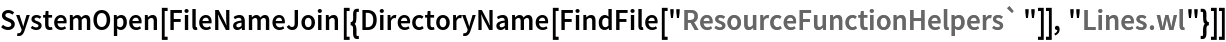Function Repository Resource:

# NormalLine

Compute properties of the normal line to a curve at a given point

Contributed by: Wolfram|Alpha Math Team
 ResourceFunction["NormalLine"][expr,{x,a},{y,b}] returns an association of properties of the normal line to expr, viewed as an equation in x and y, at the point {x,y}={a,b}. ResourceFunction["NormalLine"][expr,{x,a},{y,b},prop] returns the value of the normal line property prop. prop can also be a list of properties. ResourceFunction["NormalLine"][expr,{x,a},y] returns information relating to one, among possibly several, of the normal lines to expr at x=a. ResourceFunction["NormalLine"][expr,x,{y,b}] returns information relating to one, among possibly several, of the normal lines to expr at y=b.

## Details

Allowed values of prop are:
 "SlopeInterceptEquation" equation of the normal line in slope intercept form "StandardFormEquation" equation of the normal line in standard form "PointSlopeEquation" equation of the normal line in point slope form "Slope" slope of the normal line "PointOfNormalcy" point at which the normal line is computed "HorizontalIntercept" horizontal intercept for the normal line equation "VerticalIntercept" vertical intercept for the normal line equation "Plot" plot of the normal line equation All association of information returning all allowed properties
If expr does not have head Equal, then expr is treated as an expression defining y in terms of x. In other words, ResourceFunction["NormalLine"][expr,{x,a},y,] is equivalent to ResourceFunction["NormalLine"][y==expr,{x,a},y,] if expr has a head other than Equal.
If only one coordinate of the intersection point is given, the other coordinate is inferred. For expressions that are multivalued at the given value of x or y, information on only one of potentially several normal lines is returned.

## Examples

### Basic Examples (2)

Compute the slope-intercept equation of the normal line to a curve at a given point:

 In:=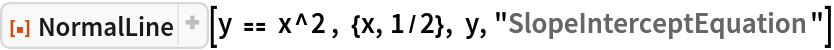Out=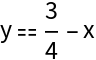Visualize this result:

 In:=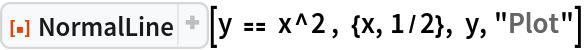Out=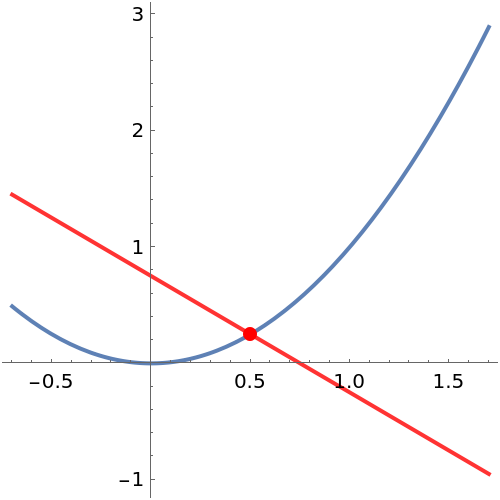Compute the slope of this normal line:

 In:=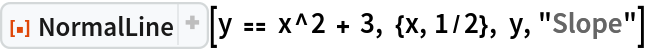Out=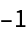Compute the horizontal intercept of this normal line:

 In:=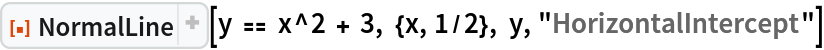Out=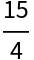Get the standard-form equation of this normal line:

 In:=Out=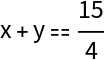Get an Association of properties of a normal line to a curve:

 In:=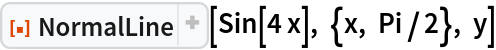Out=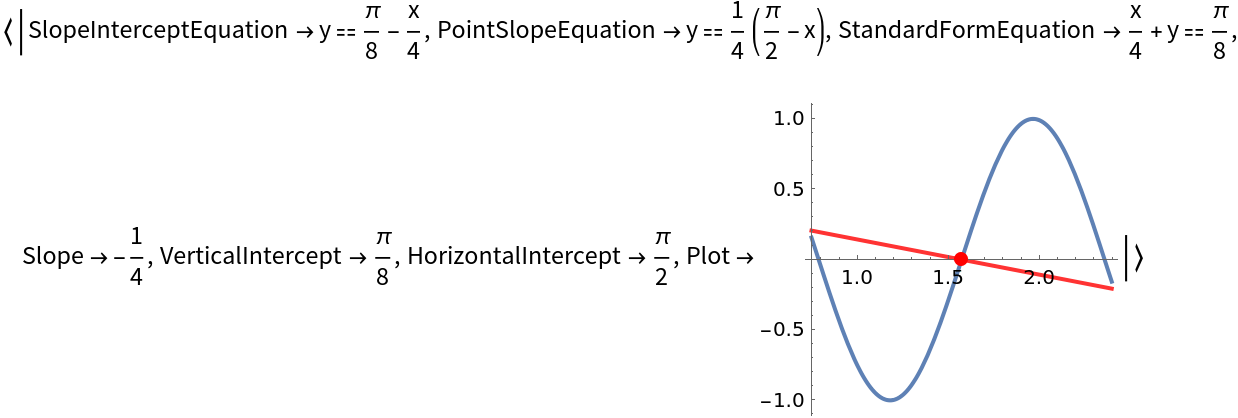Get just the point-slope equation of this normal line:

 In:=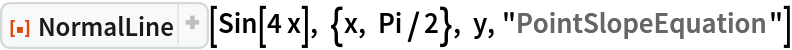Out=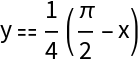### Scope (1)

The first argument to NormalLine can be an implicit definition of a curve:

 In:=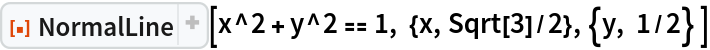Out=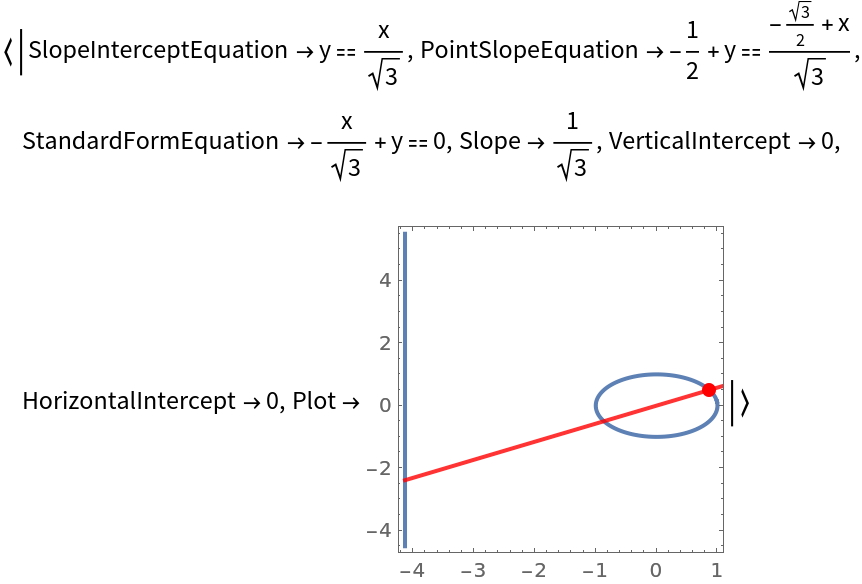### Properties and Relations (2)

If a normal line is parallel to a coordinate axis, its intercept with that axis is None:

 In:=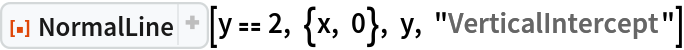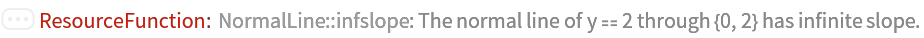Out=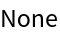Requesting normal line information about a point that is not on the curve will result in an error message:

 In:=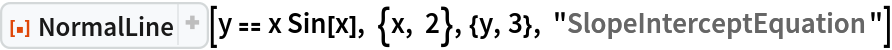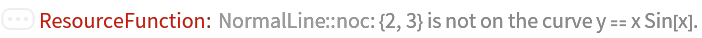Out=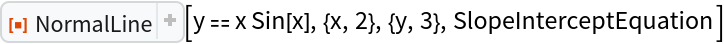### Possible Issues (3)

If one coordinate is not specified, information on only one of the possible normal lines at the given coordinate value is returned:

 In:=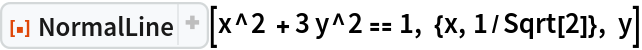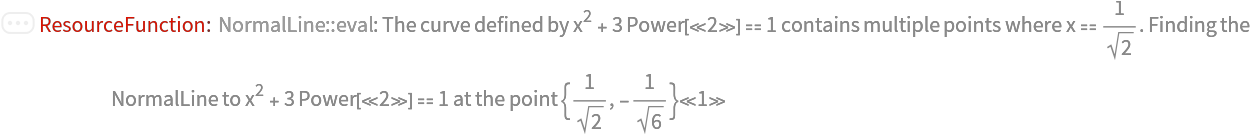Out=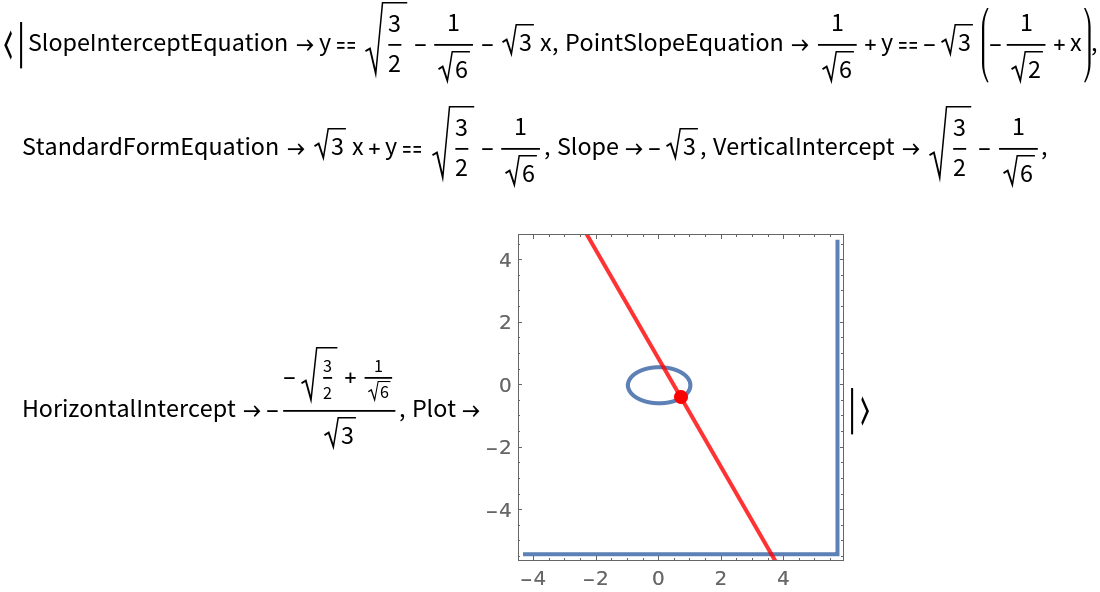Vertical normal lines have infinite slope. Some of their properties may not be defined:

 In:=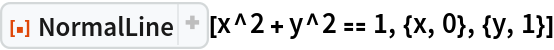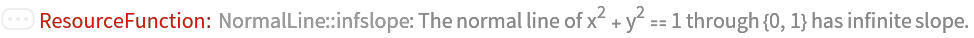Out=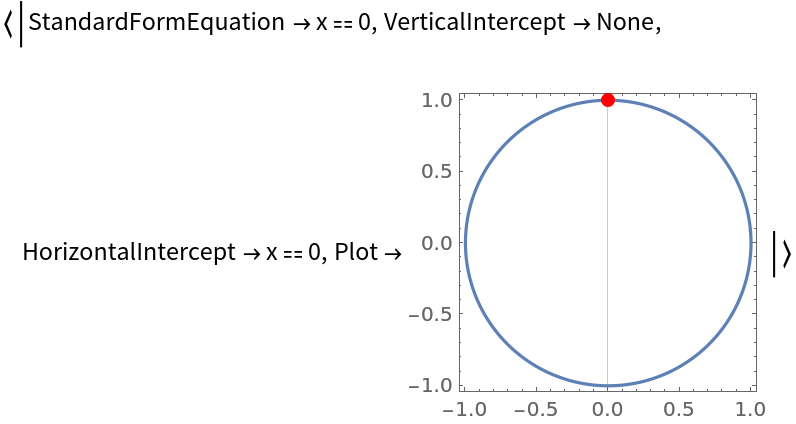If a function has a cusp or a discontinuity at the given point, no normal line is returned:

 In:=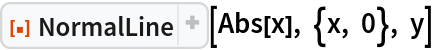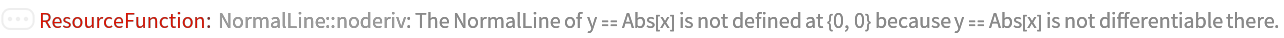Out=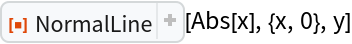## Publisher

Wolfram|Alpha Math Team

## Version History

• 3.1.0 – 04 August 2023
• 3.0.0 – 23 March 2023
• 2.0.1 – 29 March 2022
• 2.0.0 – 26 May 2021
• 1.0.0 – 08 March 2021

## Author Notes

To view the full source code for TangentAndNormalLine, evaluate the following:

 In:=